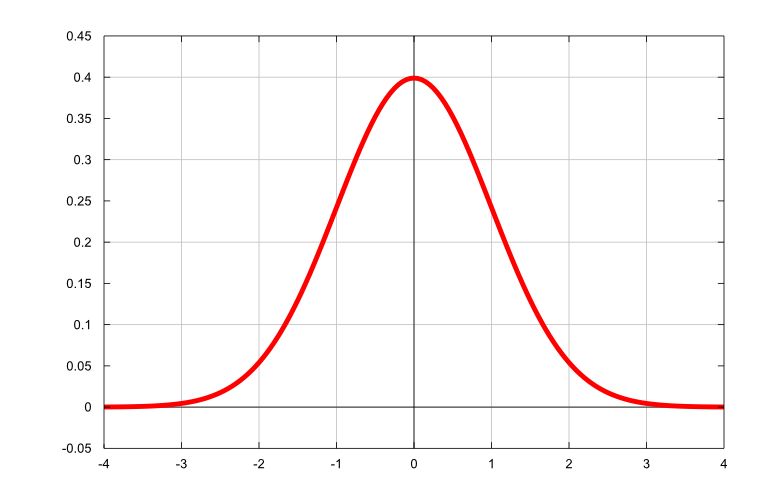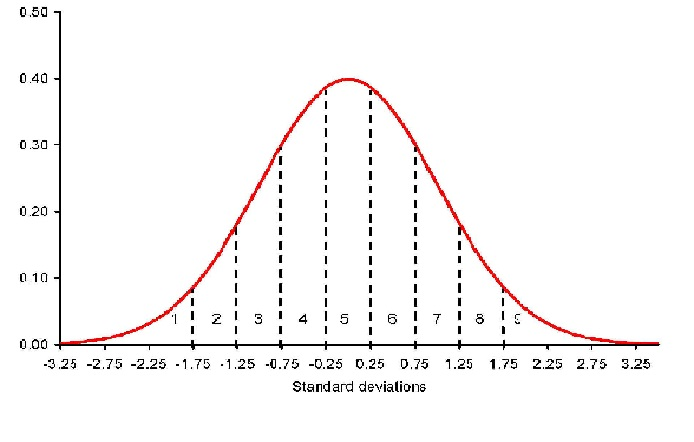# Difference between Gaussian and Normal Distribution

In order to analyze a set of data, many statistical tests are applied to make some general conclusions, and the most commonly used test in this regard is the Normal/Gaussian distribution. Because of  its central limit theorem, this distribution plays are very important role in statistics. There is basically no difference between the two; the Gaussian and the normal distribution are the two names of the same thing.

The normal distribution is called Gaussian distribution because the person who discovered it was Carl Friedrich Gauss. Another name of normal or Gaussian distribution is the bell shaped distribution because when it is plotted on a graph its shape resembles a bell.

This is a continuous distribution and the probability distribution function for a random variable ‘a’ in case of Normal/Gaussian distribution is such that P(a)= [1/( σ√2π)  e^(-(a-µ)2/2σ^2 )  where σ is the standard deviation and µ is the mean (it can also be a median or mode). Here ‘a’ falls between -∞ to +∞ i.e the Gaussian distribution ranges from negative infinity to positive infinity.

This distribution belongs to the elliptical distribution class. It is symmetric about x = μ; the mean, median and mode are considered same and are denoted by µ. In addition to this, the value of the skewness and kurtosis is 0 in case of Normal/Gaussian distribution.

Central limit theorem states that the distribution of means will still be a Gaussian distribution even if the samples are sufficiently large and the population is not Gaussian.

Another important property of this distribution is that it is the limiting form of binomial distribution. In case of the binomial distribution if p is finite and N is very large then we get a continuous variable and hence the binomial distribution becomes the Normal/Gaussian distribution.

There are different versions of normal distributions but their basic form remains the same. For instance the standard normal distribution is another case of normal distribution in which µ=0 and σ=σ^2=1. The benefit is the ease of reference to the standardized tables in order to solve the probability density functions and cumulative distributions.

### Instructions

• 1

Normal Distribution:

A normal distribution is defined as a probability distribution that plots the values of the results symmetrically about the mean of the probability.

Image Courtesy: statlect.com• 2

Gaussian Distribution:

This is also known as the normal distribution and is the normal curve of a theoretical frequency distribution with finite mean and variance.•